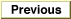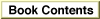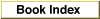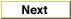# Legacy DocumentImportant: The information in this document is obsolete and should not be used for new development.

Inside Macintosh: QuickDraw GX Environment and Utilities /
Chapter 8 - QuickDraw GX Mathematics / QuickDraw GX Mathematics Reference
Mathematical Functions / Vector Operations

### VectorMultiply

You can use the `VectorMultiply` function to obtain the dot product of two vectors with 64-bit accuracy.

```wide *VectorMultiply(long count, const long *vector1, long
step1, const long *vector2, long step2, wide *dot);
```
`count`
The size of each vector.
`vector1`
A pointer to one of the two vectors.
`step1`
The index increment for the `vector1` vector.
`vector2`
A pointer to the second of two vectors.
`step2`
The index increment for the `vector`2 vector.
`dot`
A pointer to the destination of the result.
function result
A pointer to the dot product of the two vectors.
##### DESCRIPTION
The `VectorMultiply` function calculates the `wide` dot product of the parameters `vector1` and `vector2`. The size of each vector is given by the `count` parameter. The index increment is given by the parameters `step1` and `step2`, respectively. The `dot` parameter points to the destination `wide` number and is returned as the function result.

Examples of how to use the `VectorMultiply` function are provided in the section "Performing Vector Operations" beginning on page 8-29.